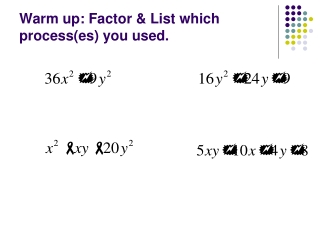DownloadDownload PresentationWarm up: Factor & List which process(es) you used.

# Warm up: Factor & List which process(es) you used.

Télécharger la présentation## Warm up: Factor & List which process(es) you used.

- - - - - - - - - - - - - - - - - - - - - - - - - - - E N D - - - - - - - - - - - - - - - - - - - - - - - - - - -
##### Presentation Transcript

1. Warm up: Factor & List which process(es) you used.

2. Homework Questions? • Pg. 664 # 14 – 31

3. 12-1 Simplifying Rational Expressions Objective: To simplify rational expressions. Standard 12.0

4. Excluded Values Excluded Values: values that make the denominator zero (because we cannot divide by zero). Always find the excluded values BEFORE you cancel anything out. Examples: State the excluded values

5. Steps • Factor top and bottom of fraction separately • GCF • Binomial: Difference of 2 squares • Trinomial: X-Box, Perfect Square trinomial • Find excluded values (denominator 0) • Cancel out factors (what top and bottom have in common)

6. Example 1 Excluded values:

7. Homework • Simplifying Fractions worksheet

8. Math Lab Warm up: Factor

9. Steps • Factor top and bottom of fraction separately • GCF • Binomial: Difference of 2 squares • Trinomial: X-Box, Perfect Square trinomial • Find excluded values (denominator 0) • Cancel out factors (what top and bottom have in common)

10. Excluded Values Excluded Values: values that make the denominator zero (because we cannot divide by zero). Always find the excluded values BEFORE you cancel anything out. Examples: State the excluded values